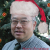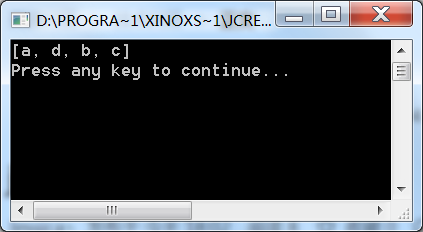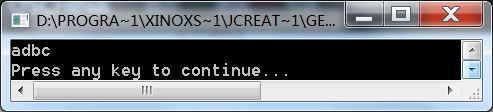# java有没有类似指针的集合

Jessica丶 发布于 04/08 18:02

0``````import java.util.*;
public class Test{

public static void main(String[] args) {
String a []={"a","b","c"};
String s ="abc";
List<String> list = new ArrayList<String>();
for (int i=0;i<a.length;i++)
list.add(a[i]);
list.add(list.indexOf("b"),"d");
System.out.println(list);
}
}  ````````````public class Test{

public static void main(String[] args) {
String s ="abc";
String result = s.substring(0,s.indexOf('b')) + 'd'+s.substring(s.indexOf('b'));
System.out.println(result);
}
}  ``````